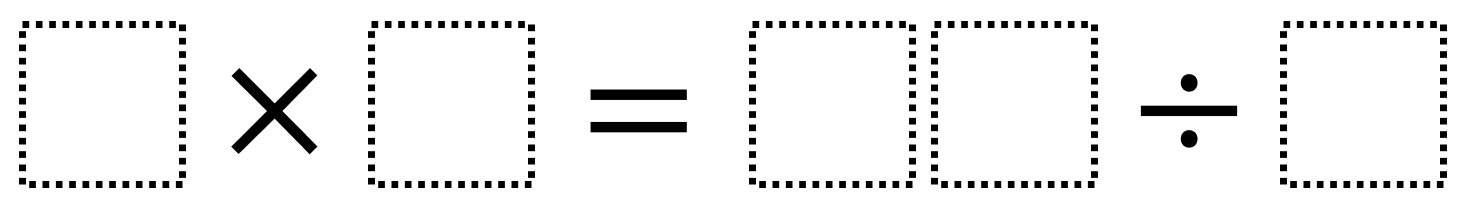# Multiply and Divide Within A Hundred 2

Directions: Using the digits 2 to 9 at most one time each, place a digit in each box to make a correct equations where the value is as close to 38 as possible.### Hint

What digits would be better worse to divide by? What are the greatest values you can make on the division side?

The closest you can get to 38 is 7 x 6 = 84 ÷ 2 (which equals 42 on both sides).

Source: Robert Kaplinsky

## Multiply and Divide Within A Hundred 1

Directions: Using the digits 2 to 9 at most one time each, place a digit …

1.6 X 8 = 192/4

••and you watch coco melon

•your should be… you’re as in you are

On another note, let’s not put people down.

Be kind!

2.•you cant divide by 1 because they say 2-9